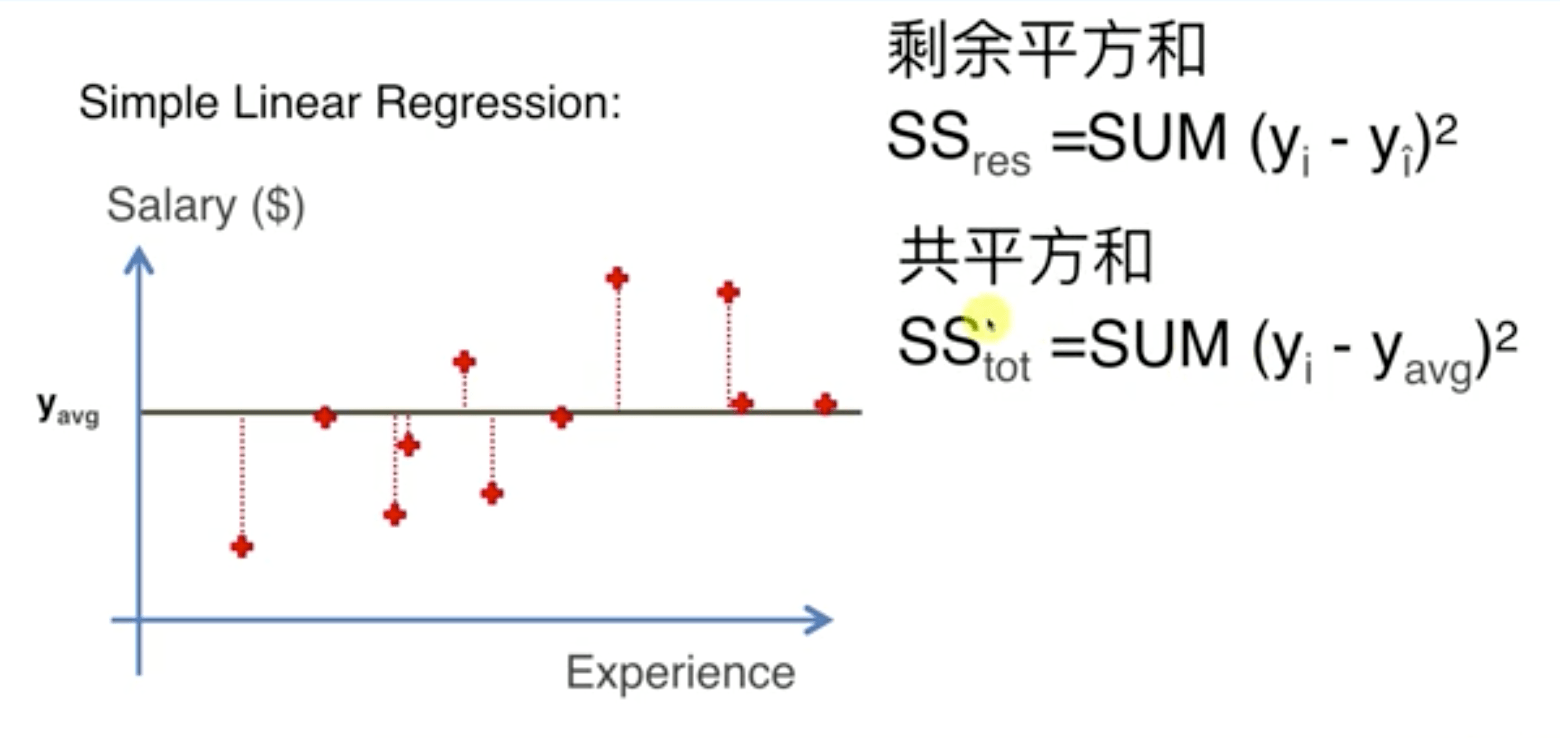# 机器学习A-Z～评估回归模型的表现

## R平方R^2 = 1 - \frac{SS_{res}}{SS_{tot}}

Adj R^2 = 1 - (1 - R^2) * \frac{n-1}{n-p-1}